# Dim Sum Classifier – from Data to App part 2

Picture Credits here

In the previous post, we see how we can acquire data, process, clean and train an Image Classifier to identify some yummy dim sums.

In this post, we shall look at completing the loop by developing the web app using starlette (a framework similar to that of flask but supports asynchronous IO), setting up and automating deployment of our web app with Github, Docker contanier and Render.

The very helpful fast.ai course team and community has given us a quick start with the following resources:

In part 1, we used learn.export to export a file named export.pkl (no surprises there). We will need to host it in a cloud service separately. One reason is that model files are typically large files (in our case its 98MB) that usually do not work well with Github unless using extensions like Git LFS. One benefit is that we do not need to redeploy our docker image when we update the model file.

For this app, we shall be using Google Drive as the hosting service. As per the deployment guide, we shall use this Google Drive Direct Link Generator. Doing this enables the model file to be downloaded bypassing the intermediate download prompts.

### Customizing and testing the web app

Using the aforementioned quick start template, we need to customized the application for our classifier. Below are some of the notable changes:

1. Update the model’s direct link in export_file_url

This will be the link generated previously.

1. Update classes labels

You can get this list by using the .classes method of ImageDataBunch (data.classes line in previous post).

To provide a nicer output with Chinese characters, the labels were updated accordingly as below.

classes = ['char siu sou (叉烧酥)', 'chee cheong fun (猪肠粉)', 'har gow (虾饺)', 'lo bak go (萝卜糕)', 'siu mai (烧卖)']

1. Change prediction to output numerical values

A corresponding change is made to the line below to output the numerical values so as to reference the above labels.

prediction = classes[int(learn.predict(img))]


Before running the app locally, we need to ensure the dependencies are installed by executing the command:

pip install -r requirements.txt


If your environment already has the fastai environment set up, you can just install the outstanding packages like starlette, uvicorn etc

Run the app locally by executing the command below and open localhost:5000 in your browser:

python app/server.py serve


You can see the outputs of the local instance below.

Once tested, make sure you push your changes to github for deployment later. Remember to remove the model file from the models/ folder.

### Setting up your Render account and deploying the app

Using the Render service gives us some benefits like application cloud hosting and CI/CD capabilities like auto rebuilding and deploying of Docker containers upon commits into target branch.

After verifying your email and logging into the account, Select New Web Service from the Services page

IN the next page, click on Connect Github to grant Render access.

After signing into your Github account, you will need to choose your account and select your target repository. In this case, we choose the personal account and grant Render access to only the dimsum_classifier_fastai repository.

After linking your repository, setup the web service configuration. In this case the name is selected as dimsum_fastai (this will be your subdomain) and Environment selected is Docker, which will use the Dockerfile within the repository to build the container image. Ensure that you are choosing the correct branch, here it is master. With no credit card information, only the Starter plan can be chosen.

After clicking on Create Web Service, you will see a command line screen showing the build process (building of docker container, installing dependencies, pushing image to container registry, setup of web service etc). Wait for the process to finish (you will see the status turn to ‘live’)

Once live, you will be able to access the app via https://<subdomain name>.onrender.com. In our case, it will be https://dimsum-fastai.onrender.com (note that the underscore has been changed to hyphen).

Below are the images when accessing the live link.

### Stop (Suspend) & Delete Web Service

To take down the web service after testing, you can delete or suspend the the web service. In your service, click on settings and scroll to the bottom. There you can select either Delete Web Service or Suspend Web Service. Please see the images below.

### Takeaways

We demonstrated in 2 posts the entire life cycle from data acquisition to application and model deployment. Some elements can be improved upon to make the app more 12-factor compliant like managing the configurations like the model configurations in the environment and to convert the prediction functionality into another REST API.

Code links can be found below:

# Dim Sum Classifier – from Data to App part 1

Picture Credits here

In a typically machine learning lifecycle, we will need to acquire data, process data, train and validate/test models and finally deploy the trained models in applications/services. In this first part of two post, inspired by fast.ai 2019 lesson 2, we shall build a Dim Sum (a Cantonese bite-size style of cuisine with many yummy choices) classifier application by leveraging on Google Images as a data source. Due to the wide variety of choices, we shall focus on 5 common dim sum dishes below, with links for your interest:

The image links are curated with gi2ds (Google Image Search to Dataset), a very convenient JavaScript snippet created by Christoffer Björkskog. Details can be found at the blog link here and on Github.

After using the tool, 5 text files with 200 image links corresponding to each of the dishes are prepared and saved in Google Drive for import into Google Colab, which will be used for processing and training.

We shall proceed to setup the Google Colab environment, import the file containing image links from Google Drive and download the images using the download_images function. Fast.AI library also provides a very handy verify_images function that helps you to check for valid images and prunes off files that cannot be used.

!curl -s https://course.fast.ai/setup/colab | bash

Updating fastai...
Done.

%reload_ext autoreload
%matplotlib inline

from fastai.vision import *

dirs_dimsum = ['hargow', 'siumai', 'charsiusou', 'cheecheongfun','lobakgo']
files_dimsum = ['urls_hargow200.txt', 'urls_siumai200.txt',
'urls_charsiusou200.txt', 'urls_cheecheongfun200.txt',
'urls_lobakgo200.txt']

from google.colab import drive
drive.mount('/content/gdrive', force_remount=True)
root_dir = '/content/gdrive/My Drive/'
base_dir = root_dir + 'fastaiv3/'

from shutil import copy2
path = Path('data/dimsum')
for folder, file in list(zip(dirs_dimsum, files_dimsum)):
dest = path/folder
dest.mkdir(parents=True, exist_ok=True)
copy2(base_dir+'dimsum_class/'+file, dest)


path.ls()

[PosixPath('data/dimsum/charsiusou'),
PosixPath('data/dimsum/siumai'),
PosixPath('data/dimsum/hargow'),
PosixPath('data/dimsum/lobakgo'),
PosixPath('data/dimsum/cheecheongfun')]

path = Path('data/dimsum')
for folder, file in list(zip(dirs_dimsum, files_dimsum)):

classes = dirs_dimsum

for c in classes:
print(c)
verify_images(path/c, delete=True, max_size=200)


### Training the model

Once we have our dataset, we will use the ImageDataBunch.from_folder method to load the images from the folder and preview the images.

np.random.seed(42)
data = ImageDataBunch.from_folder(path, train='.', valid_pct=0.2,
ds_tfms=get_transforms(), size=224,
num_workers=4).normalize(imagenet_stats)

data.classes

['charsiusou', 'cheecheongfun', 'hargow', 'lobakgo', 'siumai']

data.show_batch(rows=3, figsize=(7,8))


From the batch, it seems that they are fine. There is one mislabeled char siu sou (叉烧酥) but we leave it for now since a small level of noisy data does not typically affect the model much. We are using transfer learning with the ResNet 34 model.

data.classes, data.c, len(data.train_ds), len(data.valid_ds)

(['charsiusou', 'cheecheongfun', 'hargow', 'lobakgo', 'siumai'], 5, 738, 184)
learn = cnn_learner(data, models.resnet34, metrics=error_rate)

Downloading: "https://download.pytorch.org/models/resnet34-333f7ec4.pth" to /home/yoke/.cache/torch/checkpoints/resnet34-333f7ec4.pth
100%|██████████| 87306240/87306240 [00:03<00:00, 24127371.58it/s]

learn.fit_one_cycle(10)

epoch train_loss valid_loss error_rate time
0 1.892826 1.363298 0.516304 00:07
1 1.473497 0.831715 0.282609 00:07
2 1.158775 0.728580 0.244565 00:07
3 0.939784 0.627291 0.217391 00:07
4 0.785094 0.622544 0.211957 00:07
5 0.670714 0.568230 0.157609 00:07
6 0.598721 0.547424 0.163043 00:07
7 0.527969 0.552321 0.173913 00:07
8 0.476596 0.549852 0.179348 00:07
9 0.443554 0.550588 0.179348 00:07
learn.save('stage-1')


After 10 epochs, we have an error rate (1-Accuracy) of 17.9% and proceed to save the model.

### Finetuning the model

We shall now fine tune the model by unfreezing the pre-trained layers for training, along with using the learning rate finder to find optimal learning rates.

learn.unfreeze()

learn.lr_find()

LR Finder is complete, type {learner_name}.recorder.plot() to see the graph.

learn.recorder.plot()

learn.fit_one_cycle(5, max_lr=slice(5e-4, 1e-3))

epoch train_loss valid_loss error_rate time
0 0.296187 0.695414 0.211957 00:07
1 0.390351 1.626430 0.309783 00:08
2 0.425384 2.619203 0.429348 00:08
3 0.367741 0.679681 0.168478 00:08
4 0.326681 0.530172 0.146739 00:08

After fine-tuning, the error rate drops to 14.6%. We shall save this model and use ClassificationInterpretation to examine the models top losses and confusion matrix.

learn.save('stage-2')

interpret = ClassificationInterpretation.from_learner(learn)

interpret.plot_confusion_matrix()

interpret.plot_top_losses(9, figsize=(15,11))


From the top losses, it seems that there are several images that are composite pictures that confuses the model, since we are only predicting one class per picture. We can use the ImageCleaner widget to view and cleanse the dataset of unwanted pictures.

Unfortunately, Google Colab does not support ipywidgets and hence we need to run some portions of the notebook on a local runtime, which is described in the follow section. We shall zip the images and saved models for download.

!zip -r download_colab.zip /content/data/dimsum


## Run in local runtime for ImageCleaner

This section to be run only locally aims to prune misleading data and labels.

Please note that DatasetFormatter does not differentiate train/validation set, hence we need to load the images using DataBlock API with explicit command to not split into training and validation set.

%reload_ext autoreload
%matplotlib inline

from fastai.vision import *

#local setup rerun
path = Path('content/data/dimsum')
np.random.seed(42)
db = (ImageList.from_folder(path)
.split_none()
.label_from_folder()
.transform(get_transforms(), size=224)
.databunch()
)

db.show_batch(rows=3, figsize=(7,8))

learn_cleaning = cnn_learner(db, models.resnet34, metrics=error_rate)

learn_cleaning.load('stage-2')

from fastai.widgets import *

ds, idxs = DatasetFormatter().from_toplosses(learn_cleaning)

ImageCleaner(ds, idxs, path)

HBox(children=(VBox(children=(Image(value=b'\xff\xd8\xff\xe0\x00\x10JFIF\x00\x01\x01\x01\x00d\x00d\x00\x00\xff…

Button(button_style='primary', description='Next Batch', layout=Layout(width='auto'), style=ButtonStyle())

<fastai.widgets.image_cleaner.ImageCleaner at 0x7f7590297860>

Below is a screenshot of the widget when running locally.

From the cleaning exercise, quite a few images have been cleaned up. Typically invalid images are:

• Expected char siu sou (叉烧酥) but images are char siu bao (叉烧包), another dim sum type or the char siu roasted meat (叉烧) itself
• composite images with irrelevant images
• Animated images

### Training the model, Round 2

After running the image cleaner, a cleaned.csv will be generated. Upload this file to Google Colab, reload and then retrain the model. Please note that no images have been deleted, hence we need to reload the cleaned data using the cleaned.csv file as reference.

!mv /content/cleaned.csv /content/data/dimsum

np.random.seed(42)

data2 = ImageDataBunch.from_csv(path, folder=".", valid_pct=0.2,
csv_labels='cleaned.csv',
ds_tfms=get_transforms(),
size=224,
num_workers=4).normalize(imagenet_stats)

data2.classes, data2.c, len(data2.train_ds), len(data2.valid_ds)

(['charsiusou', 'cheecheongfun', 'hargow', 'lobakgo', 'siumai'], 5, 472, 118)

learn_cleaned = cnn_learner(data2, models.resnet34, metrics=error_rate)

learn_cleaned.fit_one_cycle(10)

epoch train_loss valid_loss error_rate time
0 1.958007 1.473040 0.644068 00:05
1 1.535733 0.663816 0.161017 00:05
2 1.177153 0.491802 0.152542 00:05
3 0.915417 0.515325 0.152542 00:05
4 0.747979 0.513231 0.169492 00:05
5 0.633824 0.520064 0.169492 00:05
6 0.544484 0.516060 0.169492 00:05
7 0.475155 0.514972 0.169492 00:05
8 0.413666 0.512496 0.169492 00:05
9 0.370536 0.515782 0.169492 00:05

After 10 epochs, the error rate dropped by 1% as compared to the first part of training before finetuning in round 1.

### Finetuning the model – Round 2

We then proceed with finetuning the model in round 2.

learn_cleaned.unfreeze()

learn_cleaned.lr_find()

LR Finder is complete, type {learner_name}.recorder.plot() to see the graph.

learn_cleaned.recorder.plot()

learn_cleaned.fit_one_cycle(5, max_lr=slice(1e-4, 1e-3))

epoch train_loss valid_loss error_rate time
0 0.150190 0.461228 0.169492 00:05
1 0.122265 0.665217 0.177966 00:05
2 0.118568 0.567386 0.152542 00:05
3 0.104063 0.560797 0.127119 00:05
4 0.088834 0.486471 0.127119 00:05

After finetuning, the error rate is now at 12.7% as compared to that of 14.6% before cleaning. It is also noted that validation loss much higher than training loss – likely to improve with more data.

We can examine the model with ClassificationIntepretation again.

learn_cleaned.save('stage-3')

interpret_cleaned = ClassificationInterpretation.from_learner(learn_cleaned)

interpret_cleaned.plot_confusion_matrix()

interpret_cleaned.most_confused()

[('siumai', 'cheecheongfun', 4),
('lobakgo', 'cheecheongfun', 3),
('charsiusou', 'cheecheongfun', 2),
('cheecheongfun', 'hargow', 2),
('lobakgo', 'siumai', 2),
('hargow', 'cheecheongfun', 1),
('siumai', 'charsiusou', 1)]

interpret_cleaned.plot_top_losses(9, figsize=(15,11))


### Try with ResNet 50

We can also attempt with a larger model like ResNet 50.

learn_cleaned50 = cnn_learner(data2, models.resnet50, metrics=error_rate)

learn_cleaned50.fit_one_cycle(10)

epoch train_loss valid_loss error_rate time
0 1.655776 1.074644 0.423729 00:07
1 1.060038 0.426286 0.144068 00:06
2 0.765334 0.462426 0.161017 00:06
3 0.602831 0.449330 0.144068 00:06
4 0.478973 0.428820 0.127119 00:06
5 0.397765 0.433457 0.118644 00:06
6 0.332555 0.437746 0.127119 00:06
7 0.288448 0.437288 0.135593 00:06
8 0.253740 0.432407 0.135593 00:06
9 0.220242 0.432829 0.135593 00:06
learn_cleaned50.unfreeze()

learn_cleaned50.lr_find()

LR Finder is complete, type {learner_name}.recorder.plot() to see the graph.

learn_cleaned50.recorder.plot()

learn_cleaned50.fit_one_cycle(5, max_lr=slice(1e-4, 5e-3))

epoch train_loss valid_loss error_rate time
0 0.109317 0.682296 0.169492 00:07
1 0.277890 2.939289 0.262712 00:07
2 0.331545 2.263224 0.245763 00:08
3 0.309646 0.597230 0.127119 00:08
4 0.264014 0.429430 0.127119 00:07

After training and finetuning, the same error rate, which isn’t much of a surprise but validation loss is smaller and smaller difference between train and validation loss.

learn_cleaned50.export('export.pkl')

interpret_cleaned50 = ClassificationInterpretation.from_learner(learn_cleaned50)

interpret_cleaned50.plot_confusion_matrix()

interpret_cleaned50.most_confused()

[('siumai', 'hargow', 3),
('siumai', 'lobakgo', 3),
('cheecheongfun', 'hargow', 1),
('cheecheongfun', 'lobakgo', 1),
('cheecheongfun', 'siumai', 1),
('hargow', 'charsiusou', 1),
('hargow', 'cheecheongfun', 1),
('lobakgo', 'charsiusou', 1),
('lobakgo', 'cheecheongfun', 1),
('siumai', 'charsiusou', 1),
('siumai', 'cheecheongfun', 1)]


### Takeaways

In this post we created an image classifier for dim sums using google images as data source. We have also demonstrated transfer learning, ImageCleaner widget and model export using the fast.ai library. We shall be using the exported model for deployment in a web application in our next and final part – part 2.

The corresponding notebook can be found here for your review in Google Colab. One thing to note is that the image data acquired might not be fully reproducible since some links might expire.

# Rock, paper, scissors – vision transfer learning with fast.ai

Picture Credits: Wikipedia

In the previous post, we used the Rock, Paper Scissors notebook that trained a custom image classification model from scratch.

While the notebook is demonstrates building custom layers, for such a task, we can also leverage on Transfer Learning using models trained on similar image classification tasks that can often reduce time in training and experimentation and yet achieve results fairly good results, which will be shown here using the fastai v1 library as demonstrated by Jeremy Howard in his awesome Practical Deep Learning for Coders 2019 course.

For such a task, we can also leverage on Transfer Learning using models trained on similar image classification tasks that can often reduce time in training and experimentation and yet achieve results fairly good results, which will be shown here using the fastai v1 library as demonstrated by Jeremy Howard in his awesome Practical Deep Learning for Coders 2019 course.

As per last post, we start with the same dataset, using Google Colab. We shall also be updating the fastai library version in Colab and then importing the fast.ai vision module and the accuracy metric.

!curl -s https://course.fast.ai/setup/colab | bash

Updating fastai...
Done.

!wget --no-check-certificate \
-O /tmp/rps.zip

!wget --no-check-certificate \
-O /tmp/rps-test-set.zip

--2019-07-11 14:30:57--  https://storage.googleapis.com/laurencemoroney-blog.appspot.com/rps.zip
HTTP request sent, awaiting response... 200 OK
Length: 200682221 (191M) [application/zip]
Saving to: ‘/tmp/rps.zip’

/tmp/rps.zip        100%[===================>] 191.38M   125MB/s    in 1.5s

2019-07-11 14:30:59 (125 MB/s) - ‘/tmp/rps.zip’ saved [200682221/200682221]

HTTP request sent, awaiting response... 200 OK
Length: 29516758 (28M) [application/zip]
Saving to: ‘/tmp/rps-test-set.zip’

/tmp/rps-test-set.z 100%[===================>]  28.15M  93.4MB/s    in 0.3s

2019-07-11 14:31:01 (93.4 MB/s) - ‘/tmp/rps-test-set.zip’ saved [29516758/29516758]

import os
import zipfile

local_zip = '/tmp/rps.zip'
zip_ref = zipfile.ZipFile(local_zip, 'r')
zip_ref.extractall('/tmp/')
zip_ref.close()

local_zip = '/tmp/rps-test-set.zip'
zip_ref = zipfile.ZipFile(local_zip, 'r')
zip_ref.extractall('/tmp/')
zip_ref.close()

!mv /tmp/rps /tmp/train
!mv /tmp/rps-test-set/ /tmp/valid

from fastai.vision import *
from fastai.metrics import error_rate


We shall then set the batch size (bs) to 64 and load the data using the ImageDataBunch.from_folder method. This method helps to easily load training and validation datasets that are stored in separate sub-folders. This method also performs data augmentation as defined by the get_transforms() function. Last but not least, since we are using a pre-trained model, we need to normalize the data using imagenet_stats that is used by ResNet34 model (shown later). We shall then preview a small batch of the data and the data classes.

bs = 64

data = ImageDataBunch.from_folder('/tmp', ds_tfms=get_transforms(), size=224, bs=bs).normalize(imagenet_stats)

data.show_batch(rows=3, figsize=(7,6))

print(data.classes)
len(data.classes),data.c

['paper', 'rock', 'scissors']

(3, 3)

### Training the model

We shall now call the cnn_learner method that downloads the specified pre-trained model (ResNet 34) upon first-time use and trains shows the accuracy metric. You can also see the structure of ResNet 34 using learn.model. Finally, we kick start the learning process using learn.fit_one_cycle for 4 epochs using the one cycle policy that enables training with very high learning rates.

learn = cnn_learner(data, models.resnet34, metrics=accuracy)

Downloading: "https://download.pytorch.org/models/resnet34-333f7ec4.pth" to /root/.cache/torch/checkpoints/resnet34-333f7ec4.pth
100%|██████████| 87306240/87306240 [00:00<00:00, 113309789.33it/s]

learn.model

Sequential(
(0): Sequential(
(0): Conv2d(3, 64, kernel_size=(7, 7), stride=(2, 2), padding=(3, 3), bias=False)
(1): BatchNorm2d(64, eps=1e-05, momentum=0.1, affine=True, track_running_stats=True)
(2): ReLU(inplace)
(3): MaxPool2d(kernel_size=3, stride=2, padding=1, dilation=1, ceil_mode=False)
(4): Sequential(
(0): BasicBlock(
(conv1): Conv2d(64, 64, kernel_size=(3, 3), stride=(1, 1), padding=(1, 1), bias=False)
(bn1): BatchNorm2d(64, eps=1e-05, momentum=0.1, affine=True, track_running_stats=True)
(relu): ReLU(inplace)
(conv2): Conv2d(64, 64, kernel_size=(3, 3), stride=(1, 1), padding=(1, 1), bias=False)
(bn2): BatchNorm2d(64, eps=1e-05, momentum=0.1, affine=True, track_running_stats=True)
)
(1): BasicBlock(
(conv1): Conv2d(64, 64, kernel_size=(3, 3), stride=(1, 1), padding=(1, 1), bias=False)
(bn1): BatchNorm2d(64, eps=1e-05, momentum=0.1, affine=True, track_running_stats=True)
(relu): ReLU(inplace)
(conv2): Conv2d(64, 64, kernel_size=(3, 3), stride=(1, 1), padding=(1, 1), bias=False)
(bn2): BatchNorm2d(64, eps=1e-05, momentum=0.1, affine=True, track_running_stats=True)
)
(2): BasicBlock(
(conv1): Conv2d(64, 64, kernel_size=(3, 3), stride=(1, 1), padding=(1, 1), bias=False)
(bn1): BatchNorm2d(64, eps=1e-05, momentum=0.1, affine=True, track_running_stats=True)
(relu): ReLU(inplace)
(conv2): Conv2d(64, 64, kernel_size=(3, 3), stride=(1, 1), padding=(1, 1), bias=False)
(bn2): BatchNorm2d(64, eps=1e-05, momentum=0.1, affine=True, track_running_stats=True)
)
)
(5): Sequential(
(0): BasicBlock(
(conv1): Conv2d(64, 128, kernel_size=(3, 3), stride=(2, 2), padding=(1, 1), bias=False)
(bn1): BatchNorm2d(128, eps=1e-05, momentum=0.1, affine=True, track_running_stats=True)
(relu): ReLU(inplace)
(conv2): Conv2d(128, 128, kernel_size=(3, 3), stride=(1, 1), padding=(1, 1), bias=False)
(bn2): BatchNorm2d(128, eps=1e-05, momentum=0.1, affine=True, track_running_stats=True)
(downsample): Sequential(
(0): Conv2d(64, 128, kernel_size=(1, 1), stride=(2, 2), bias=False)
(1): BatchNorm2d(128, eps=1e-05, momentum=0.1, affine=True, track_running_stats=True)
)
)
(1): BasicBlock(
(conv1): Conv2d(128, 128, kernel_size=(3, 3), stride=(1, 1), padding=(1, 1), bias=False)
(bn1): BatchNorm2d(128, eps=1e-05, momentum=0.1, affine=True, track_running_stats=True)
(relu): ReLU(inplace)
(conv2): Conv2d(128, 128, kernel_size=(3, 3), stride=(1, 1), padding=(1, 1), bias=False)
(bn2): BatchNorm2d(128, eps=1e-05, momentum=0.1, affine=True, track_running_stats=True)
)
(2): BasicBlock(
(conv1): Conv2d(128, 128, kernel_size=(3, 3), stride=(1, 1), padding=(1, 1), bias=False)
(bn1): BatchNorm2d(128, eps=1e-05, momentum=0.1, affine=True, track_running_stats=True)
(relu): ReLU(inplace)
(conv2): Conv2d(128, 128, kernel_size=(3, 3), stride=(1, 1), padding=(1, 1), bias=False)
(bn2): BatchNorm2d(128, eps=1e-05, momentum=0.1, affine=True, track_running_stats=True)
)
(3): BasicBlock(
(conv1): Conv2d(128, 128, kernel_size=(3, 3), stride=(1, 1), padding=(1, 1), bias=False)
(bn1): BatchNorm2d(128, eps=1e-05, momentum=0.1, affine=True, track_running_stats=True)
(relu): ReLU(inplace)
(conv2): Conv2d(128, 128, kernel_size=(3, 3), stride=(1, 1), padding=(1, 1), bias=False)
(bn2): BatchNorm2d(128, eps=1e-05, momentum=0.1, affine=True, track_running_stats=True)
)
)
(6): Sequential(
(0): BasicBlock(
(conv1): Conv2d(128, 256, kernel_size=(3, 3), stride=(2, 2), padding=(1, 1), bias=False)
(bn1): BatchNorm2d(256, eps=1e-05, momentum=0.1, affine=True, track_running_stats=True)
(relu): ReLU(inplace)
(conv2): Conv2d(256, 256, kernel_size=(3, 3), stride=(1, 1), padding=(1, 1), bias=False)
(bn2): BatchNorm2d(256, eps=1e-05, momentum=0.1, affine=True, track_running_stats=True)
(downsample): Sequential(
(0): Conv2d(128, 256, kernel_size=(1, 1), stride=(2, 2), bias=False)
(1): BatchNorm2d(256, eps=1e-05, momentum=0.1, affine=True, track_running_stats=True)
)
)
(1): BasicBlock(
(conv1): Conv2d(256, 256, kernel_size=(3, 3), stride=(1, 1), padding=(1, 1), bias=False)
(bn1): BatchNorm2d(256, eps=1e-05, momentum=0.1, affine=True, track_running_stats=True)
(relu): ReLU(inplace)
(conv2): Conv2d(256, 256, kernel_size=(3, 3), stride=(1, 1), padding=(1, 1), bias=False)
(bn2): BatchNorm2d(256, eps=1e-05, momentum=0.1, affine=True, track_running_stats=True)
)
(2): BasicBlock(
(conv1): Conv2d(256, 256, kernel_size=(3, 3), stride=(1, 1), padding=(1, 1), bias=False)
(bn1): BatchNorm2d(256, eps=1e-05, momentum=0.1, affine=True, track_running_stats=True)
(relu): ReLU(inplace)
(conv2): Conv2d(256, 256, kernel_size=(3, 3), stride=(1, 1), padding=(1, 1), bias=False)
(bn2): BatchNorm2d(256, eps=1e-05, momentum=0.1, affine=True, track_running_stats=True)
)
(3): BasicBlock(
(conv1): Conv2d(256, 256, kernel_size=(3, 3), stride=(1, 1), padding=(1, 1), bias=False)
(bn1): BatchNorm2d(256, eps=1e-05, momentum=0.1, affine=True, track_running_stats=True)
(relu): ReLU(inplace)
(conv2): Conv2d(256, 256, kernel_size=(3, 3), stride=(1, 1), padding=(1, 1), bias=False)
(bn2): BatchNorm2d(256, eps=1e-05, momentum=0.1, affine=True, track_running_stats=True)
)
(4): BasicBlock(
(conv1): Conv2d(256, 256, kernel_size=(3, 3), stride=(1, 1), padding=(1, 1), bias=False)
(bn1): BatchNorm2d(256, eps=1e-05, momentum=0.1, affine=True, track_running_stats=True)
(relu): ReLU(inplace)
(conv2): Conv2d(256, 256, kernel_size=(3, 3), stride=(1, 1), padding=(1, 1), bias=False)
(bn2): BatchNorm2d(256, eps=1e-05, momentum=0.1, affine=True, track_running_stats=True)
)
(5): BasicBlock(
(conv1): Conv2d(256, 256, kernel_size=(3, 3), stride=(1, 1), padding=(1, 1), bias=False)
(bn1): BatchNorm2d(256, eps=1e-05, momentum=0.1, affine=True, track_running_stats=True)
(relu): ReLU(inplace)
(conv2): Conv2d(256, 256, kernel_size=(3, 3), stride=(1, 1), padding=(1, 1), bias=False)
(bn2): BatchNorm2d(256, eps=1e-05, momentum=0.1, affine=True, track_running_stats=True)
)
)
(7): Sequential(
(0): BasicBlock(
(conv1): Conv2d(256, 512, kernel_size=(3, 3), stride=(2, 2), padding=(1, 1), bias=False)
(bn1): BatchNorm2d(512, eps=1e-05, momentum=0.1, affine=True, track_running_stats=True)
(relu): ReLU(inplace)
(conv2): Conv2d(512, 512, kernel_size=(3, 3), stride=(1, 1), padding=(1, 1), bias=False)
(bn2): BatchNorm2d(512, eps=1e-05, momentum=0.1, affine=True, track_running_stats=True)
(downsample): Sequential(
(0): Conv2d(256, 512, kernel_size=(1, 1), stride=(2, 2), bias=False)
(1): BatchNorm2d(512, eps=1e-05, momentum=0.1, affine=True, track_running_stats=True)
)
)
(1): BasicBlock(
(conv1): Conv2d(512, 512, kernel_size=(3, 3), stride=(1, 1), padding=(1, 1), bias=False)
(bn1): BatchNorm2d(512, eps=1e-05, momentum=0.1, affine=True, track_running_stats=True)
(relu): ReLU(inplace)
(conv2): Conv2d(512, 512, kernel_size=(3, 3), stride=(1, 1), padding=(1, 1), bias=False)
(bn2): BatchNorm2d(512, eps=1e-05, momentum=0.1, affine=True, track_running_stats=True)
)
(2): BasicBlock(
(conv1): Conv2d(512, 512, kernel_size=(3, 3), stride=(1, 1), padding=(1, 1), bias=False)
(bn1): BatchNorm2d(512, eps=1e-05, momentum=0.1, affine=True, track_running_stats=True)
(relu): ReLU(inplace)
(conv2): Conv2d(512, 512, kernel_size=(3, 3), stride=(1, 1), padding=(1, 1), bias=False)
(bn2): BatchNorm2d(512, eps=1e-05, momentum=0.1, affine=True, track_running_stats=True)
)
)
)
(1): Sequential(
)
(1): Flatten()
(2): BatchNorm1d(1024, eps=1e-05, momentum=0.1, affine=True, track_running_stats=True)
(3): Dropout(p=0.25)
(4): Linear(in_features=1024, out_features=512, bias=True)
(5): ReLU(inplace)
(6): BatchNorm1d(512, eps=1e-05, momentum=0.1, affine=True, track_running_stats=True)
(7): Dropout(p=0.5)
(8): Linear(in_features=512, out_features=3, bias=True)
)
)

learn.fit_one_cycle(4)

epoch train_loss valid_loss accuracy time
0 0.408251 0.289994 0.879032 00:33
1 0.151992 0.190768 0.932796 00:32
2 0.078730 0.166957 0.935484 00:32
3 0.044160 0.151344 0.946237 00:32

In just 4 epochs, we can reach an accuracy of 94% as compared to the 25 epochs used in the previous notebook! We shall now have a closer look at the results using the ClassificationInterpretation method. This enable us to look at the top losses, most confused labels and the confusion matrix.

interp = ClassificationInterpretation.from_learner(learn)

losses,idxs = interp.top_losses()

len(data.valid_ds)==len(losses)==len(idxs)

True

interp.plot_top_losses(9, figsize=(15,11))


interp.plot_confusion_matrix(figsize=(12,12), dpi=60)

interp.most_confused(min_val=2)

[('scissors', 'paper', 11), ('paper', 'scissors', 6), ('paper', 'rock', 3)]

### Finetuning the model

By default, the learner created have the pre-trained model weights frozen (not changed during the 4 epochs of training) and only trains the additional neural network layer added by the cnn_learner method. We can unfreeze the pre-trained model weights for training.

learn.unfreeze()

learn.fit_one_cycle(1)

epoch train_loss valid_loss accuracy time
0 0.042922 0.574411 0.862903 00:33

It turns out that just continuing training the the model after unfreezing lowers the accuracy! To ensure that we can continue to train using optimal learning rates, we use the learn.lr_find method to find them before training again.

learn.lr_find()

LR Finder is complete, type {learner_name}.recorder.plot() to see the graph.

learn.recorder.plot()


From the learning rate plot above, it is determined that a rate between 5e-4 and 3e-3 has the "steepest" slope, indicating a good range for max learning rates.

learn.fit_one_cycle(2, max_lr=slice(5e-4,3e-3))

epoch train_loss valid_loss accuracy time
0 0.112085 0.033667 0.981183 00:33
1 0.039996 0.010633 1.000000 00:34
This time, we reached an accuracy of 100%, with the confusion matrix as below.
interp2 = ClassificationInterpretation.from_learner(learn)

losses2,idxs2 = interp2.top_losses()

len(data.valid_ds)==len(losses2)==len(idxs2)

True

interp2.plot_confusion_matrix(figsize=(12,12), dpi=60)


Hence with transfer learning and utilizing methods like one-cycle policy and learning rate finder, we can train the model with less time (less epochs) and leverage on established pre-trained models (ResNet 34) rather than training a model from scratch.

You can find the corresponding notebook for this post here.

# Serving Rock, Paper, Scissors Image Classifier App built with Tensorflow 2, Keras and Flask

Picture Credits: Pixabay

In this post, we shall be looking at serving a Tensorflow 2 Keras image classification model with a Flask app.

We shall be leveraging on the Rock Paper Scissors Tensorflow 2 Notebook created by Laurence Moroney and built on the Image Classifier App template provided by Fing on the Github repository here.

### Training and saving the Model in Google Colab

To train the model, we can run the aforementioned Jupyter Notebook on Google Colab. To train the model successfully, we will need to ensure that Tensorflow 2 beta is installed with the following command:

!pip install tensorflow-gpu==2.0.0-beta1


For convenience, you can open the notebook provided in my repository here.

In Google Colab screen above, click on File > Open Notebook. In the pop-up screen, click on the Github tab. You will see the screen below.

Copy and paste the notebook link here and click on the search button (magnifying glass icon). Once the notebook has loaded, Select ‘Runtime > Change Runtime Type’ from the menu.

At this point, please note that you need to have a Google Account and register yourself to use Google Colab. Otherwise you will note be able to execute the notebook.

You will see the Notebook settings tab. Ensure that ‘Python 3’ and ‘GPU’ options have been selected as shown below and click on Save.

Once done, you can execute the notebook by selecting Runtime > Run all from the menu.

Open the files tab in the left pane. The left pane can be expanded by clicking on the icon highlighted below.

In the files tab below, locate the model file ‘rps.h5’ and click the ‘Download’ button. Note that this same model file that has already been uploaded to the Github repository.

### Running the image classifier app

As the original template has been built with Tensorflow 1.x in mind, the code has been updated to work with the Tensorflow 2 beta 1 library.

Clone (or download and unzip) the repository here and ensure that the model has been saved inside the ‘models’ folder. (Model already provided)

$git clone https://github.com/yoke2/rps_tf2_flask_app.git  Execute the commands below to install dependencies. You will need Python 3+ to be installed. $ cd rps_tf2_flask_app/
$pip install -r requirements.txt  Run the app using the command below and open the application in your browser on http://localhost:5000 $ python app.py


You will now see the app below.

Choose a file to classify. Test files have been provided in misc/test_files for you to use.

After clicking on the ‘Predict!’ button, you will see the prediction shown as below.

You can then attempt to deploy this app in the cloud. You can also containerize the application using Docker using the provided Dockerfile in the repository.

# Find difference between CSV files using PowerShell

If you are on Windows, you can use PowerShell to find the differences between two CSV files. Sample code below:

$file1 = import-csv -Path "D:\path\to\file1.csv"$file2 = import-csv -Path "D:\path\to\file2.csv"
Compare-Object $file1$file2 -property column_to_identify_row -IncludeEqual


# Find version of python package installed

Below are 3 methods we can try to find the version of an installed python package. We shall use scipy as an example.

### Using pip

Method 1 – For pip 1.3 and above: pip show scipy

Method 2 – Alternative (works with older versions of pip): pip freeze | grep scipy

### Using version attribute

Method 3 – Launch python/ipython, then execute the commands below:

import scipy
scipy.__version__


Reference for Method 1 is here. Reference for Method 2 is here.

# Tool to read large text files

Sometimes, you might need to view and search very large text files like logs or SQL dumps. On Windows and Linux, you can give glogg a try. Please note that this application is read-only.

# Running Apache Spark with sparklyr and R in Windows

RStudio recently released the sparklyr package that allows users to connect to Apache Spark instances from R. In addition, this package offers dplyr integration, allowing you to utilize Spark as you use dplyr functions like filter and select, which is very convenient. The package will also assist you in downloading and installing Apache Spark if it is a fresh install. This post covers the local install of Apache Spark via sparklyr and RStudio in Windows 10.

As per the guide, install the latest preview release of RStudio and run the following commands to install sparklyr

install.packages("devtools")
devtools::install_github("rstudio/sparklyr")


Once installed, you should see a new tab beside Environment and History tabs in RStudio – the Spark tab. You will be able to see a “New Connection” button.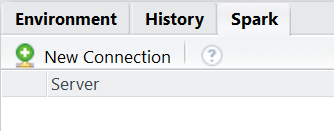On clicking the button, you will be able to set various options from Spark and Hadoop versions (at time of writing – Spark 1.6.2 and Hadoop 2.6) to connecting to local or remote Spark clusters and whether to use dplyr as the DB interface. Let’s use the defaults here.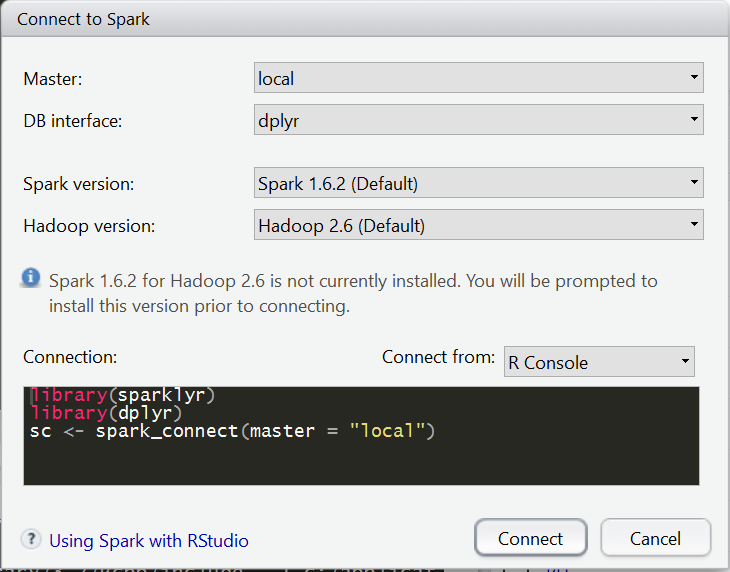If this is a fresh install, RStudio will prompt a confirmation dialog box: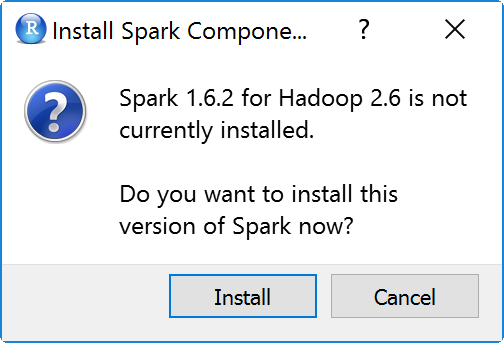Upon clicking “Install”, RStudio will then proceed to download and install Apache Spark for you and attempt to connect to the local Spark instance.

At this stage you might receive a similar error message below:

Error in start_shell(scon, list(), jars, packages) :
Failed to launch Spark shell. Ports file does not exist.
Parameters: --packages "com.databricks:spark-csv_2.11:1.3.0,com.amazonaws:aws-java-sdk-pom:1.10.34" --jars "<PATH TO R PACKAGES>\R\win-library\3.2\sparklyr\java\rspark_utils.jar"  sparkr-shell D:\Temp\RtmpO0cLos\file23c0703c73bf.out

running command '"C:\Users\<USERNAME>\AppData\Local\rstudio\spark\Cache\spark-1.6.2-bin-hadoop2.6\bin\spark-submit.cmd" --packages "com.databricks:spark-csv_2.11:1.3.0,com.amazonaws:aws-java-sdk-pom:1.10.34" --jars "<PATH TO R PACKAGES>\R\win-library\3.2\sparklyr\java\rspark_utils.jar"  sparkr-shell <PATH TO TEMP DIRECTORY>\Temp\RtmpO0cLos\file23c0703c73bf.out' had status 127


If you meet this error, it may be due to Windows security permissions of the .CMD files for Apache Spark. To resolve this issue, go to the Apache Spark install directory, which should be C:\Users\<USERNAME>\AppData\Local\rstudio\spark\Cache\spark-1.6.2-bin-hadoop2.6\bin\. You should be able to see the files ending with extension .cmd. At the time of writing, the files include

beeline.cmd
pyspark.cmd
pyspark2.cmd
run-example.cmd
run-example2.cmd
spark-class.cmd
spark-class2.cmd
spark-shell.cmd
spark-shell2.cmd
spark-submit.cmd
spark-submit2.cmd
sparkR.cmd
sparkR2.cmd


For each of the these .CMD files, edit the security permission for your USERNAME to allow “Read & execute” as shown below: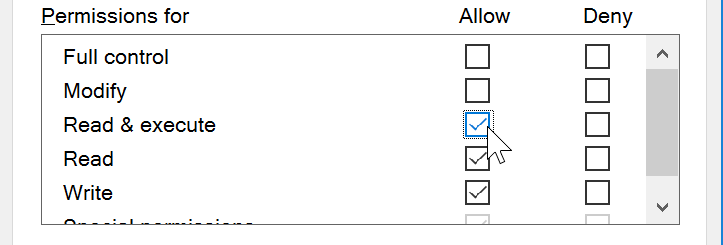After editing the permissions, when you attempt to connect to Spark by running the commands below, you should not experience any more errors.

> library(sparklyr)
> library(dplyr)
> sc <- spark_connect(master = "local")


To verify, you can try out the examples from the RStudio guide, or try the adapted example below:

> iris_tbl <- copy_to(sc, iris)
> iris_tbl
Source:   query [?? x 5]
Database: spark connection master=local app=sparklyr local=TRUE

Sepal_Length Sepal_Width Petal_Length Petal_Width Species
<dbl>       <dbl>        <dbl>       <dbl>   <chr>
1           5.1         3.5          1.4         0.2  setosa
2           4.9         3.0          1.4         0.2  setosa
3           4.7         3.2          1.3         0.2  setosa
4           4.6         3.1          1.5         0.2  setosa
5           5.0         3.6          1.4         0.2  setosa
6           5.4         3.9          1.7         0.4  setosa
7           4.6         3.4          1.4         0.3  setosa
8           5.0         3.4          1.5         0.2  setosa
9           4.4         2.9          1.4         0.2  setosa
10          4.9         3.1          1.5         0.1  setosa
# ... with more rows
>


Visually, you would be able to see the data frame in the Spark tab as well: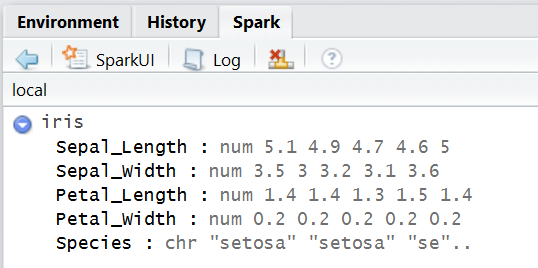You can now have fun with Apache Spark in RStudio! You might also want to add the Apache Spark install directory C:\Users\<USERNAME>\AppData\Local\rstudio\spark\Cache\spark-1.6.2-bin-hadoop2.6\bin to your path so that you can run Spark shells (sparkr,pyspark and spark-shell) in the command line. For example, with PySpark, you should see a similar welcome screen as below after the initialization messages.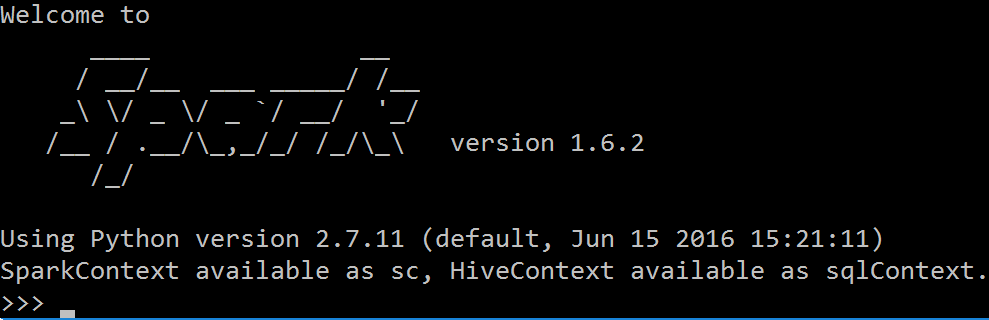# RStudio Cheat Sheets

In this resource page RStudio provides various cheatsheets for various packages that is very useful for doing data science in the R environment including data wrangling, visualization and package development.